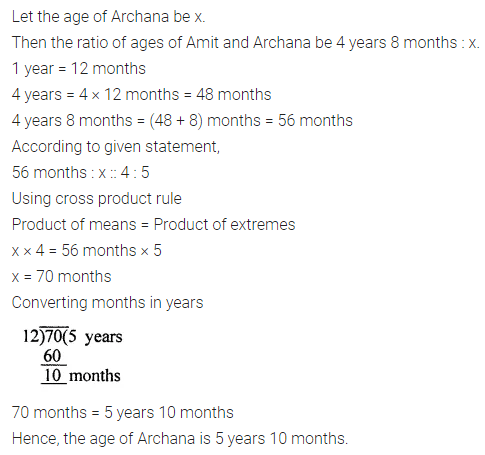# ML Aggarwal Class 7 Solutions for ICSE Maths Chapter 6 Ratio and Proportion Ex 6.2

## ML Aggarwal Class 7 Solutions for ICSE Maths Chapter 6 Ratio and Proportion Ex 6.2

Question 1.
Which of the following statements are true?
(i) 2.5 : 1.5 :: 7.0 : 4.2
(ii) $$\frac { 1 }{ 2 } :\frac { 1 }{ 3 } =\frac { 1 }{ 3 } :\frac { 1 }{ 4 }$$
(iii) 24 men : 16 men = 33 horses : 22 horses.
Solution: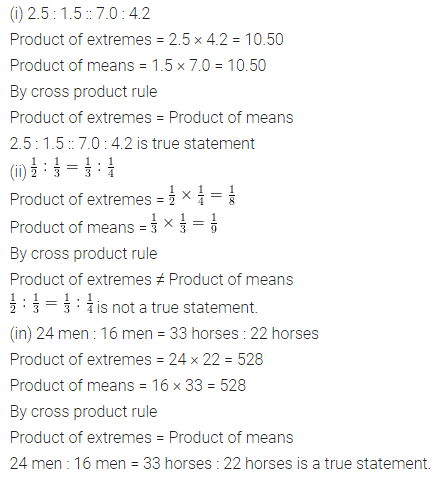Question 2.
Check whether the following numbers are in proportion or not:
(i) 18, 10, 9, 5
(ii) 3, 3$$\frac { 1 }{ 2 }$$, 4, 4$$\frac { 1 }{ 2 }$$
(iii) 0.1, 0.2, 0.3, 0.6
Solution: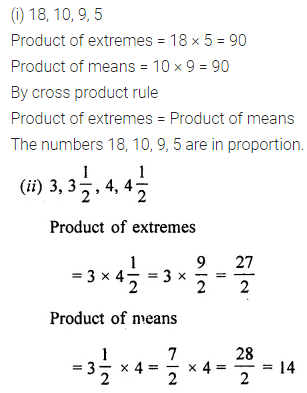Question 3.
Find x in the following proportions:
(i) x : 4 = 9 : 12
(ii) $$\frac { 1 }{ 13 }$$ : x :: $$\frac { 1 }{ 2 }$$ : $$\frac { 1 }{ 5 }$$
(iii) 3.6 : 0.4 = x : 0.5
Solution: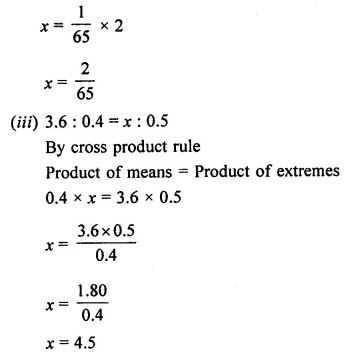Question 4.
Find the fourth proportional to
(i) 42, 12, 7
(ii) $$\frac { 1 }{ 3 }$$, $$\frac { 1 }{ 4 }$$, $$\frac { 1 }{ 5 }$$
(iii) 3 kg, 12 kg, 15 kg
Solution:Question 5.
Check whether 7, 49, 343 are in continued proportion or not.
Solution: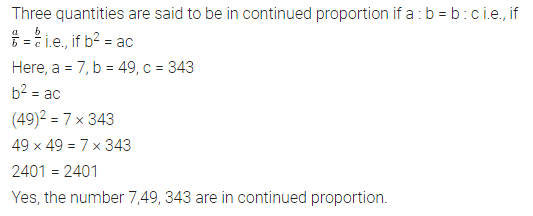Question 6.
Find the third proportional to
(i) 36, 18
(ii) 5$$\frac { 1 }{ 4 }$$, 7
(iii) 3.2, 0.8
Solution: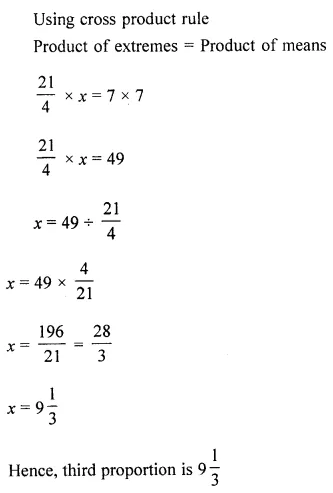Question 7.
The ratio between the length and width of a rectangular sheet of paper is 7 : 5. If the width of the sheet is 20.5 cm, find its length.
Solution: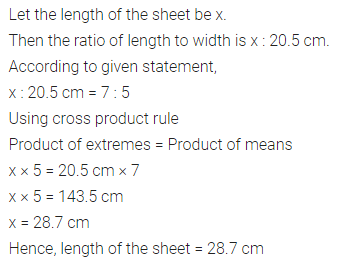Question 8.
The ages of Amit and Archana are in the ratio 4 : 5. If Amit is 4 years 8 months old, find the age of Archana.
Solution: